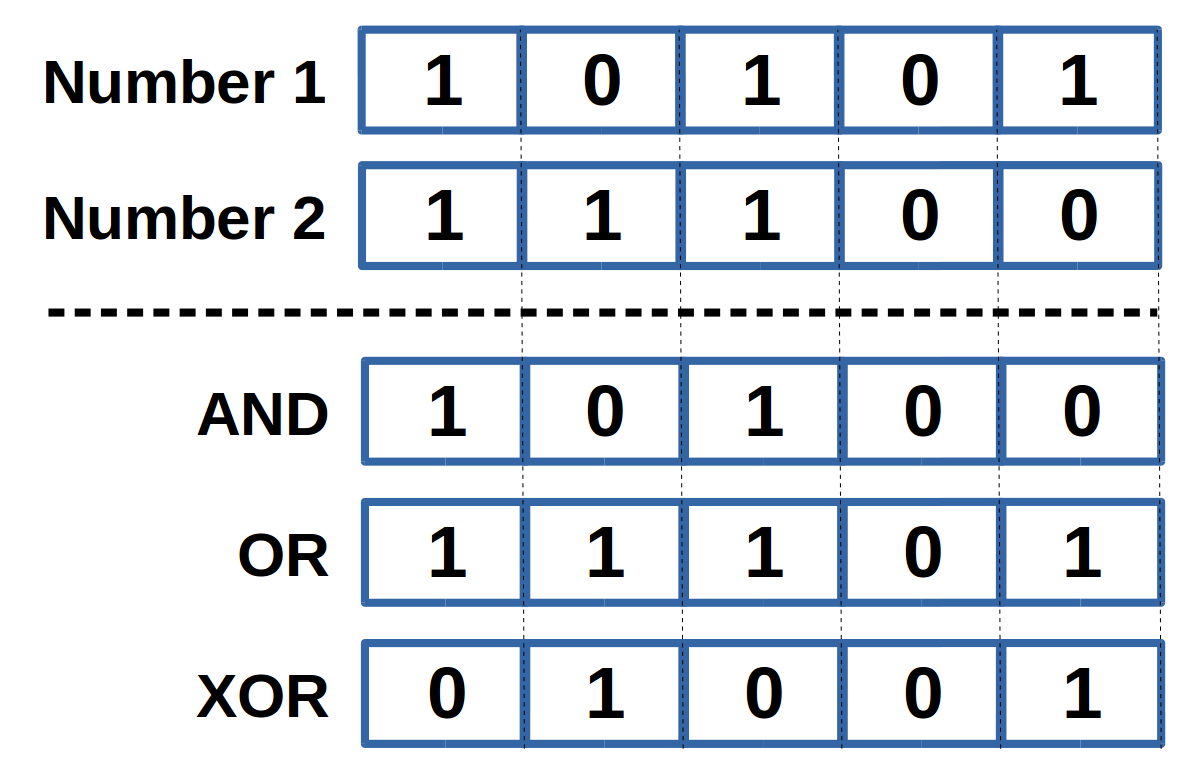# Bitwise Calculator

Created by Philip Maus
Reviewed by Steven Wooding
Last updated: Oct 11, 2022

The bitwise calculator lets you execute bitwise AND, bitwise OR, and bitwise XOR (exclusive or) operations. This tool allows you to input numbers from the binary, octal, and decimal number systems and presents the result of the bitwise operations in all those numeral systems.

## What is a bit? And what is a numeral system?

A bit is the smallest unit of information used in digital communication and electronics. Information is encoded in a logical state, which can only have two different values: 1 or 0. Alternatively, the logic pairs true/false, on/off, or yes/no can be used. Since a single bit can only encode a very limited amount of information, bits are lined up to represent larger amounts of data. For example, one byte is a succession of 8 bits, equaling 2^8 logical states.

Most of us are not used to thinking in the way of bits when talking about numerical information. So let's compare binary numbers made from bits of information to our habitual decimal system:

• The decimal system, also known as the base-10 system, uses the digits 1 to 9. Every digit of a number corresponds to a power of the base 10. For example, the number 123 can be expressed as 1*10² + 2*10¹ + 3*10⁰ (scientific notation).
• Binary numbers are founded on base 2. So every digit of a number corresponds to a power of 2. For example, the binary number 101 equals 1*2² + 0*2¹ + 1*2⁰, which equals 5 in the decimal system.

The binary converter provides a closer inside into the world of the binary system. It describes the same method used in this application to convert negative numbers from the decimal to the binary system: the signed two's complement representation.

There are other popular numeral systems besides the binary and decimal ones, namely the octal system based on the number 8 and the hexadecimal or 16-base system. You can check out our decimal to octal converter and decimal to hexadecimal converter to learn more about these number systems.

## What is a bitwise operator? AND, OR, and XOR.

To use this bitwise operator calculator, we must first understand bitwise operations.A bitwise operation is executed on a binary number, which can also be seen as a string of bits. The image displays how it works. We have two input integers in binary format, and the two binary numbers are compared bit by bit, position by position, from the first to the last. The bitwise calculator can perform 3 different bitwise operations: bitwise AND, bitwise OR, and bitwise XOR:

• Bitwise AND: The corresponding bit of the result is 1 if both input numbers have a 1 on this bit; otherwise, it is 0.
• Bitwise OR: The corresponding bit of the result is 1 if at least one input number has a 1 on this bit; otherwise, it is 0.
• Bitwise XOR/exclusive OR: The corresponding bit of the result is 1 if one and only one input number has a 1 on this bit; otherwise, it is 0.

Bitwise operations are the base for numerous real-world applications reaching from data compression and encryption over finite state machines and finding a network address from an IP address and a subnet mask (what is a subnet mask?).

## How to use the bitwise calculator?

This article introduced the concepts of bits and different numeral systems as well as the bitwise operators AND, OR, and XOR. Let us now get to know the bitwise calculator. For example, we want to find the results for a bitwise AND for the numbers 87 and 101, both in the decimal system.

1. Choose the number of bits in the binary representation. Since 8 bits allow for an input of numbers between -128 and 127 in the decimal system, this is sufficient for our cause.
2. Choose your input datatype. The bitwise operator calculator supports numbers from the binary, octal, and decimal systems. We choose decimal.
3. Input your data in fields number 1 and number 2 in the corresponding numeral system. The order of input, for us, 87 and 101, does not matter.
4. Choose your bitwise operator from the options AND, OR, and XOR. We want the AND operator.
5. The bitwise calculator presents your result as numbers from the binary, decimal, and octal systems:
• binary: 0100 0101
• octal: 105
• decimal: 69

In case your binary result could be either understood as a negative number in signed notation or a positive number in unsigned notation, both decimal system results are displayed.

That's it; now you know how to handle bitwise operations. And if you are interested in other bit operations, give our bit shift calculator a visit.

Philip Maus
Input data
Binary representation
8-bit
Datatype
Decimal
You can enter decimal numbers between -128 and 127.
Number 1
Number 2
Bitwise operation
Operation
AND
People also viewed…

### Circle Theorems

With our circle theorems calculator, you can understand all of the different circle theorems!

### Circle skirt

Circle skirt calculator makes sewing circle skirts a breeze.

### Quarter circle area

The quarter circle area calculator will find all the necessary data right when you need it.

### Titration

Use our titration calculator to determine the molarity of your solution.